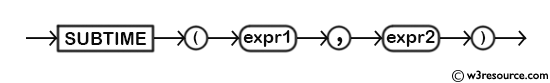# MySQL SUBTIME() function

## SUBTIME() function

MySQL SUBTIME() subtracts one datetime value from another. It allows for precise time calculations and adjustments, making it useful in various scenarios.

This function is useful in -

• SUBTIME() is valuable for making precise adjustments to time values, such as subtracting seconds, minutes, or hours.
• It supports event scheduling by calculating new times based on time intervals, which is important for scheduling tasks or events.
• The function allows for subtracting time intervals in various formats, such as hours and minutes.
• SUBTIME() can help validate and adjust time values to ensure they remain within meaningful ranges.
• The function is useful for manipulating time-based data, allowing for adjustments and transformations.
• SUBTIME() helps transform time values by subtracting or adjusting them according to specific requirements.
• SUBTIME() can be used for comparing times by subtracting one time value from another and analyzing the difference.
Syntax:
```SUBTIME(expr1,expr2)
```

Arguments:

Name Description
expr1 A datetime.
expr1 A datetime.

Syntax Diagram:MySQL Version: 8.0

Pictorial Presentation:Example: MySQL SUBTIME() function

The following statement will return a datetime value between two datetimes 2009-05-18 10:29:43.999999 and 19 3:31:18.000002 specified in the arguments.

Code:

`````` SELECT SUBTIME('2009-05-18 10:29:43.999999','19 3:31:18.000002');
```
```

Output:

``` mysql> SELECT SUBTIME('2009-05-18 10:29:43.999999','19 3:31:18.000002');
+-----------------------------------------------------------+
| SUBTIME('2009-05-18 10:29:43.999999','19 3:31:18.000002') |
+-----------------------------------------------------------+
| 2009-04-29 06:58:25.999997                                |
+-----------------------------------------------------------+
1 row in set (0.00 sec)
```

Video Presentation:

All Date and Time Functions: